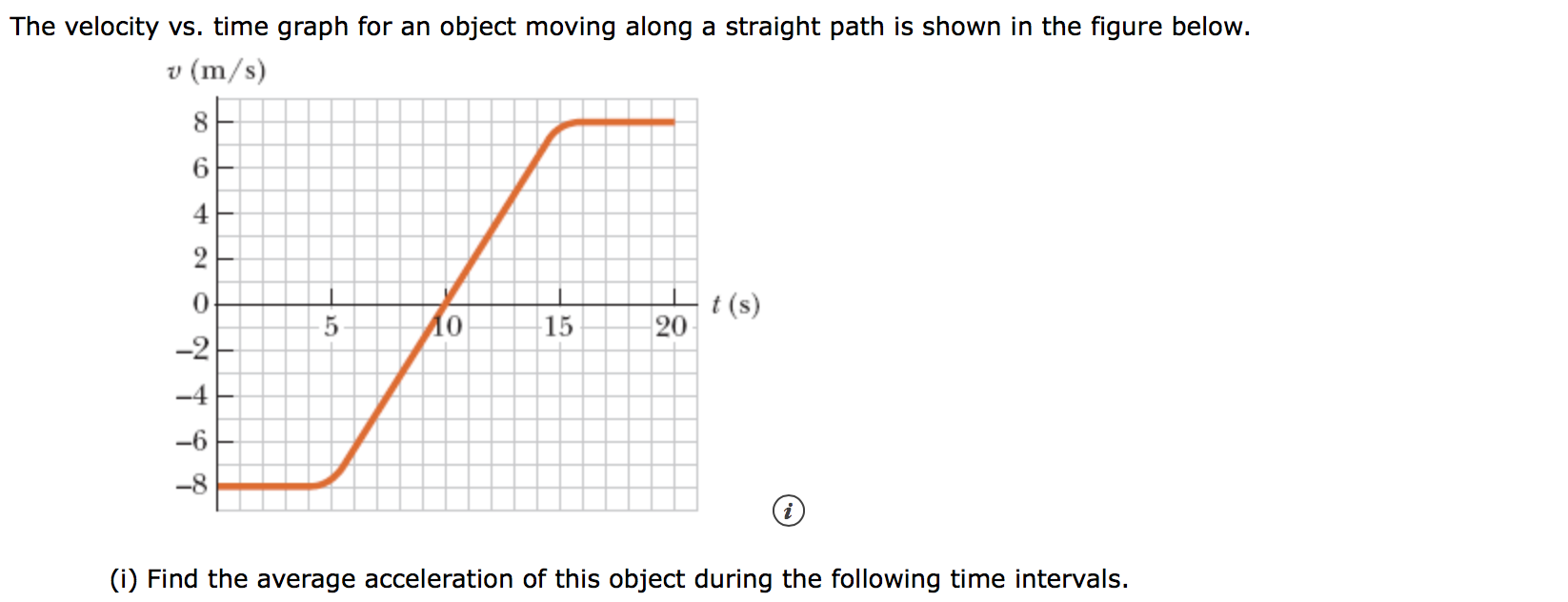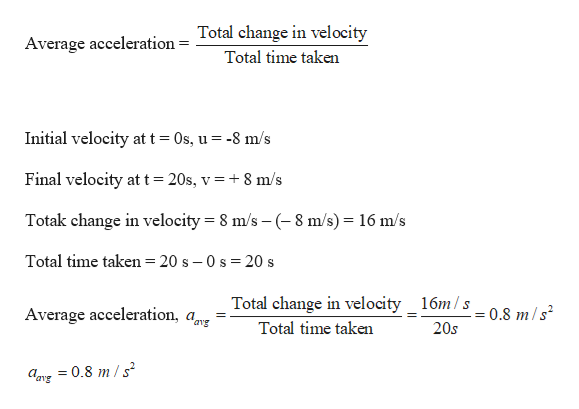# The velocity vs. time graph for an object moving along a straight path is shown in the figure below.v (m/s)8620t(s)20Ао15-2-4(i) Find the average acceleration of this object during the following time intervals.

Question

a) 0 s to 20 shelp_outlineImage TranscriptioncloseThe velocity vs. time graph for an object moving along a straight path is shown in the figure below. v (m/s) 8 6 2 0 t(s) 20 Ао 15 -2 -4 (i) Find the average acceleration of this object during the following time intervals. fullscreen
check_circle

Step 1

Given:

Step 2

Calculating the average accel...help_outlineImage TranscriptioncloseAverage acceleration = Total change in velocity Total time taken Initial velocity at t 0s, u -8 m/s Final velocity at t = 20s, v = 8 m/s Totak change in velocity 8 m/s - (8 m/s) 16 m/s Total time taken = 20 s - 0 s 20 s Total change in velocity 16m/s 0.8 m/s Average acceleration, ar avg Total time taken 20s fullscreen

### Want to see the full answer?

See Solution

#### Want to see this answer and more?

Solutions are written by subject experts who are available 24/7. Questions are typically answered within 1 hour.*

See Solution
*Response times may vary by subject and question.
Tagged in

### Kinematics